# Excel Not Sorting Numbers Correctly? Try These Fixes

This tutorial shows some possible fixes when Excel is not sorting numbers correctly.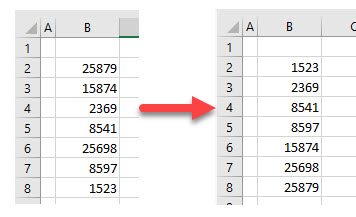There are many times in Excel that data is imported from the internet or from other programs into Excel. Often this data is not imported or copied into Excel in a consistent number format. If this is the case, it might make sorting numerically incorrect.

## Clean Function

Consider the worksheet below: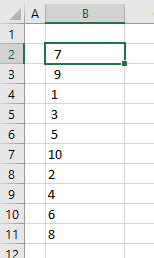1. If you try and sort this numerical list using Excel’s Sort feature, then it sorts in the order shown above!
2. Each of these numbers is actually stored as text, and some of the numbers have spaces in the cells before the numbers. To fix this, clean the data.
3. Click in the cell to the right of the first number, and then type in this formula:
``=CLEAN(B2)*1``

Using the CLEAN Function, the formula above automatically removes any invalid characters (e.g., spaces), and the *1 converts the remaining value to a number.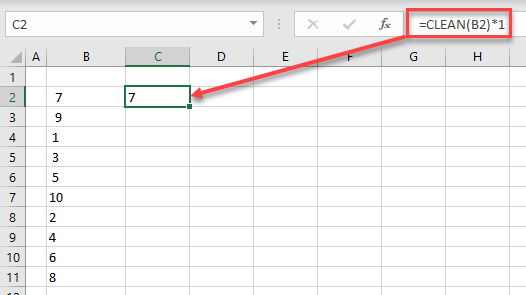You could also use the TRIM Function to remove any leading or trailing spaces, but it doesn’t remove any unprintable characters that may have been imported.

1. Now, copy the formula down to the remaining cells and then in the Ribbon, go to Home > Editing > Sort & Filter > Sort Smallest to Largest.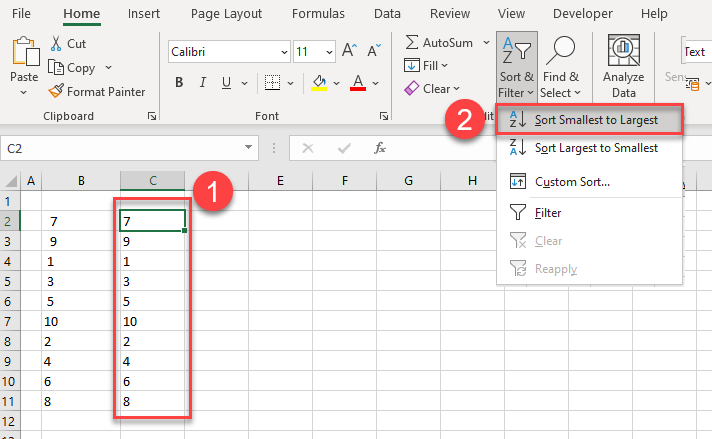The data is then sorted numerically.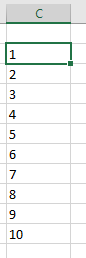## Value Function

You can also use the VALUE Function to convert your text to a number.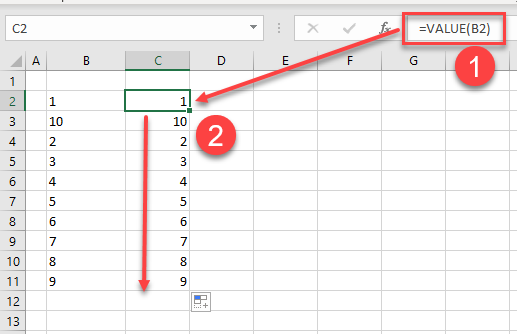1. Select the first cell to convert and then type in the formula.
``=VALUE(B2)``
1. Copy the formula down to the remaining cells. You should now be able to sort these cells correctly.
2. As your result contains a formula and you may actually need values rather than formulas for your data, copy and paste values to get rid of the formulas. First, highlight the sort range.
3. In the Ribbon, go to Home > Clipboard > Copy.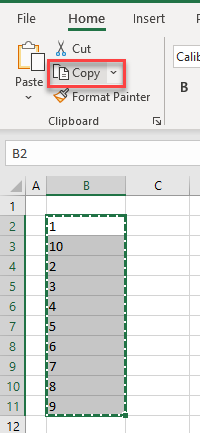1. Then, again in the Ribbon, go to Home > Clipboard > Paste > Paste Values.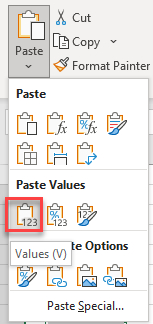1. Select Values (V).

Your formulas are now replaced by values.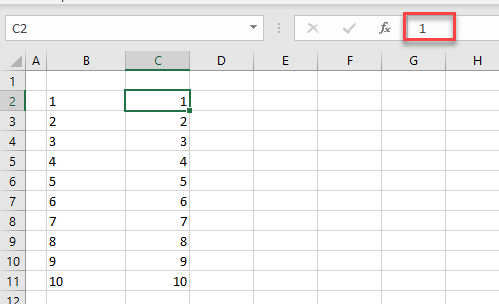## Convert to Number

Another useful fix is converting the values in the cells to a number. This is possible if your data is stored as text, but Excel recognizes that it could be a number. An error tag appears as a small green triangle in the top-left corner of each cell where this is occurring.

1. Select the cells where this is occurring and click the arrow by the small yellow triangle that appears on the right side. This shows you the error and a list of options.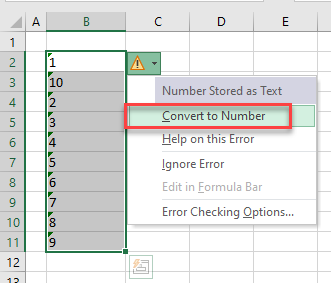1. Select Convert to Number.
2. Now, sort the numbers correctly.